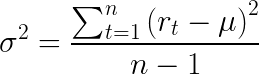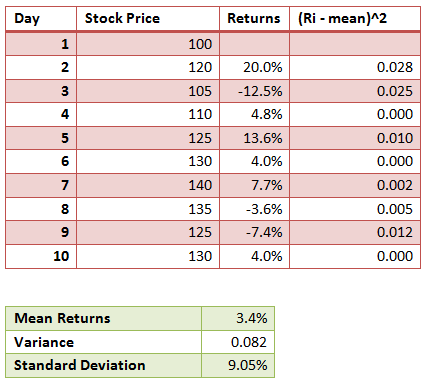# Calculate Variance and Standard Deviation of an Asset

After discussing the calculation of returns on investments, let’s now learn about how to measure the risks associated with these returns.

In general, the risk of an asset or a portfolio is measured in the form of the standard deviation of the returns, where standard deviation is the square root of variance. Let’s look at how standard deviation and variance is calculated.

The variance is calculated as follows. Once you have the price data, the first step is to calculate the returns. What kind of returns we have depends on the periodicity of the data. For example, if we have daily prices, then we calculate the daily returns, which is calculated as (P(t1) – P(t0))/p(t0).

Using the returns data, we calculate the mean/average returns. The variance of the asset returns will then be the average of square of the difference between the returns and the mean.Where n is the number of observations in the data, Rt represents the returns for each time period and μ represents the mean returns.

The standard deviation will simply be the square root of the variance.

The following is a simple example that illustrates the calculation:Get our Data Science for Finance Bundle for just $29$51.